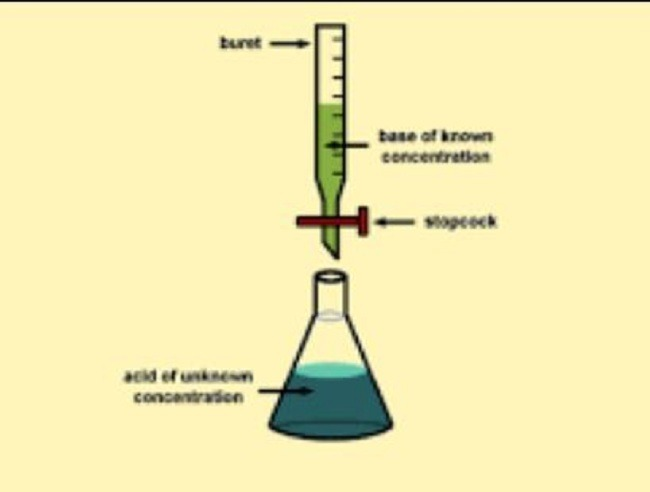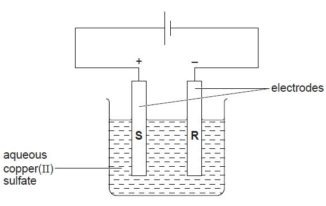January 16, 2022WAEC Chemistry Practical Specimen Questions and Answers for 14th September 2021 is Out

WAEC Chemistry Practical Specimen Questions and Answers& Titration for 14th September 2021 is Out

Are you searching for Waec chemistry practical Answers for 14th September 2021? Then you are at the right place.

Our WAEC Waec chemistry practical Answers and Expo for 14th September 2021 is absolutely free and will be updated 1:30 mins before the start of the examination.

The Waec chemistry practical 2021 paper for SSCE will now be written on Tuesday 14th September 2021 from 9:30 am to 11:30 am for the 1st set and 12:00 am to 2:00 pm for the 2nd set. The paper is Waec Chemistry practical alternative A.

We made this post to show apparatus, Waec chemistry practical specimen, graphs and calculations that are used in the Chemistry Practical Examination questions and syllabus.

Here, we will be providing you with the Waec Chemistry practical 2021 questions and materials that will be used for Examination preparation. Read answers below.WAEC Chemistry Practical 2021 Apparatus.

The following apparatus and materials will be required by each candidate for waec chemistry practical 2021;
(a) one burette of 50cm³ capacity.
(b) one pipette, either 20cm³ or 25cm³.
(c) the usual apparatus for titration.

WAEC Chemistry Practical Specimen Questions and Answers for 14th September 2021 is Out

(d) the usual apparatus and reagents for qualitative work including the following with all reagents appropriately labelled;
(i) dilute sodium hydroxide solution.
(ii) dilute hydrochloric acid.
(iii) dilute trioxonitrate(v) acid
(iv) silver trioxonitrate(v) solution.
(v) acidified potassium dichromate solution.
(vi) aqueous ammonia.
(vii) lime water.
(viii) red and blue litmus paper.
(ix) dilute tetraoxosulphate(vi) acid.

(e) Spatula
(f) filtration apparatus.
(g) one beaker
(h) one boiling tube
(i) four test tubes.
(j) Methyl orange as an indicator.
(k) mathematical table/calculator
(l) wash bottle containing distilled/deionized water.
(m) a burning splint
(n) watch glass
(o) bunsen burner/source of heat
(p) droppers
(ix) dilute tetraoxosulphate (vi) acid.

READ ALSO:  WAEC Physics Essay & OBJ Questions and Answers for 15th September 2021
• 150cm³ of a solution of HCL, in a corked flask or bottle, labelled “An”. These should all be the same containing 9.0cm³ of concentrated HCL (about 60%) per dm³ of solution.
• 150cm³ of NaOH solution in a corked flask or bottle labelled “Bn”. These should all be the same containing 4.8g of NaOH per dm³ of solution.
• one spatula of a uniform mixture of CUSO4 and (NH4)2CO3 in a specimen bottle and corked immediately, and labelled “Cn”. The components of the mixture should be in the ratio of 1:1 by mass.
• One spatula-full of crystals of iodine in a specimen bottle labelled “Dn”. This must be the same for all candidates.

WAEC Chemistry Practical Specimen Questions and Answers for 14th September 2021 is Out

1. A is 0.100 mol dm-3 solution of an acid. B is a solution NaOH containing 2.8 g per 500 cm3.
(a) Put A into the burette and titrate it against 25.0 cm3 portions B using methyl orange as an indicator. Repeat the titration to obtain consistent titres.  Tabulate your readings and calculate the average volume of A used.

(b) From your results and the information provided above, calculate the:
(i) number of moles of acid in the average titre;
(ii) number of moles of NaOH in the volume of B pipetted;
(iii) mole ratio of acid to base in the reaction
[H = 1.00, O = 16.0, K = 39.0]

(b) (i) number of moles of acid = 0.100 x VA
1000
=   X mole(s) [3sig. Fig to score]

1000cm3 contains 0.100 mole(s)
VA will contain 0.100 x VA
1000
=   X moles [3 Sig. Fig. to score]

(ii) Number of moles of NaOH in B
500cm3 of B contains 2.8g of NaOH
1000cm3 of B will contain 2.8 x 1000 = 5.6 NaOH
500
Molar mass of NaOH = 39 + 16 + 1 or 56 gmol-1

READ ALSO:  WAEC Assures Students Affected With COVID-19

Conc of B = 5.6  = 0.100 mol dm-3
56

(iii) Mole ratio of acid to base = X: Y to nearest whole number ratio.

2. C is a mixture of two salts. Carry out the following exercises on C. Record your observations and identify any gas(es) evolved. State the conclusion drawn from the result of each test.

(a) Put all of C into a boiling tube and add about 5cm3 of distilled water.  Stir thoroughly and filter. Keep both the residue and the filtrate.

(b) To about 2 cm3 of the filtrate, add few drops of K2Cr2O7/H+. Boil the mixture and then allow to cool as (D).

(c) (i) Put the residue in a test tube and add dilute NaOH(aq). Shake the mixture and divide the solution into two portions.
(ii) To the first portion from (c)(i), add NaOH(aq) in drops and then in excess.
(d) What organic class does C belong to?

ANS:

 TEST OBSERVATION INFERENCE a)  C+ burning splint C burns when ignited with a burning splint. C is flammable. b) (i) C + Water C forms a solution with water. C is a mixture with water. (ii) C + K2Cr2O7/H+ve + Heat No visible reaction. The K2Cr2O7/H+ve changes colour to green. C is a reducing agent. c)  Residue from b (D) + C + NaOH(aq) D dissolves to form a reddish-brown solution. A yellowish precipitate is formed and an antiseptic smell is given out. D is a solution in D Ethanol, ethanal or a secondary alkanol present. d) C C belongs to Alkanols

3. (a) Explain briefly the observations in each of the following processes:
(i) when carbon(IV) oxide is bubbled through lime water, it turns milky but the milkiness disappears when the gas is bubbled for a long time;
(ii) a precipitate of calcium hydroxide is insoluble in excess sodium hydroxide solution whereas that of lead (II) hydroxide is soluble.

(b) (i) What is a primary standard solution?
(ii) Calculate the mass of sodium trioxocarbonate(V) required to prepare 250 cm3 of 0.15 mol dm-3 solution.
[Na = 23.0; O = 16.0; C = 12.0]

(c) Name one gas that can be collected by:
(i) upward displacement of air;
(ii) downward displacement of air.

WAEC Chemistry Practical Specimen Questions and Answers for 14th September 2021

ANS: (a) (i): Insoluble CaCO3 formation is responsible for the milkiness produce when CO2 is bubbled through lime water while the disappearance of milkiness is due to the formation of soluble Ca(HCO3)2.
Lime water turns milky with CO2 because  CaCO3/ CaCO3(s)  is formed. Milkiness disappear when excess CO2 reacts with CaCO3 in water medium forming the soluble Ca(HCO3)2/ Ca(HCO3)2 (aq).
(ii) Calcium hydroxide is not amphoteric. Does not react with an alkali NaOH whereas lead (II) hydroxide is amphoteric so reacts with excess NaOH.

(b) (i) Primary standard solution is one whose concentration is known and can be used to standardize another solution.
(ii)    M (Na2CO3)    =  106 gmol-1
m(Na2CO3)     =  C x M x V
=   0. 15 x 106 x 0. 25
=   3. 98 g

(c) (i) Carbon(IV) oxide, sulphur (IV) oxide, hydrogen chloride, oxygen, nitrogen (IV) oxide, chlorine, hydrogen sulphide.
(ii) Ammonia, oxygen, hydrogen, methane.PS: Once again, there is nothing like waec chemistry expo. Do not fall victim to scammers online trying to obtain money from you with fake promises of having access to live question paper before the exam. What we have on this page are likely exam questions from waec past questions and answers to serve as a revision guide.Yabacampus Team

View all posts by Yabacampus Team →